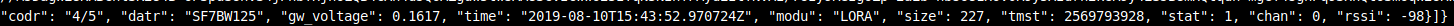# Adding information to _make_node_packet(...)

• I am currently setting up a lorawan nanogateway ( in preparation for my pygate) .
The nanogateway is implemented on a Lopy4, using the default `nanogateway.py` code supplied by pycom.

My use case does however require that I use solar to power the gateway and nodes. Currently the setup is working smoothly, however I have no way to inspect and send the gateway voltage via Lora > TTN > DB

I have tried modifying the nanogateway code as follows:

``````from machine import ADC
...
def __init__(self):
...
self.voltage_pin = ADC().channel(pin='P18', attn=ADC.ATTN_11DB)
...

...

def get_battery_voltage(self):
# Get pin 18 voltage using voltage devider
R1 = 47*1000
R2 = 100*1000
battery = self.voltage_pin.voltage()*(R1 + R2)/R2
return battery/1000
...

def _make_node_packet(self, rx_data, rx_time, tmst, sf, bw, rssi, snr):
bat = self.get_battery_voltage()
RX_PK["rxpk"]["time"] = "%d-%02d-%02dT%02d:%02d:%02d.%dZ" % (rx_time, rx_time, rx_time, rx_time, rx_time, rx_time, rx_time)
RX_PK["rxpk"]["tmst"] = tmst
RX_PK["rxpk"]["freq"] = self._freq_to_float(self.frequency)
RX_PK["rxpk"]["datr"] = self._sf_bw_to_dr(sf, bw)
RX_PK["rxpk"]["rssi"] = rssi
RX_PK["rxpk"]["lsnr"] = snr
RX_PK["rxpk"]["data"] = ubinascii.b2a_base64(rx_data)[:-1]
RX_PK["rxpk"]["size"] = len(rx_data)
RX_PK["rxpk"]["gw_voltage"] = bat
return ujson.dumps(RX_PK)
``````

Conceptually this works well as I receive gw_voltage in my terminal over pybytes:(Battery not connected value expected to be <0V)

However gw_voltage is not present on TTN.

How can I go about fixing this? Or is there an alternative approach?
Thanks!
Re: lorawan nanogateway pytrack gps